# Frequency Changing and Tracking in Receivers

## Frequency Changing and Tracking in Receivers:

The mixer is a nonlinear device having two sets of input terminals and one set of output terminals. The signal from the antenna or from the preceding RF amplifier is fed to one set of input terminals, and the output of the local oscillator is fed to the other set. As was know earlier, such a nonlinear circuit will have several frequencies present in its output, including the difference between the two input frequencies in AM this was called the lower sideband. The difference frequency here is the intermediate Frequency Changing and is the one to which the output circuit of the mixer is tuned.

The most common types of mixers are the bipolar transistor, FET, dual-gate metal-oxide-semiconductor field-effect transistor (MOSFET) and integrated circuit. All are generally self-excited in domestic receivers, so that the device acts as both oscillator and mixer. When tubes were common, the pentagrid and triode-hexode were made specially for self-excited mixer duty. At UHF and above, crystal (i.e., silicon) diodes have been used as mixers since before World War II, because of their low noise figures. These and other diodes, with even lower noise figures, are still used, as we will see in the microwave chapters. Naturally, diode mixers are separately excited.

### Conversion transconductance:

It will be recalled that the coefficient of nonlinearity of most nonlinear resistances is rather low, so that the IF output of the mixer will be very low indeed unless some preventive steps are taken. The usual step is to make the local oscillator voltage quite large, 1 V rms or more to a mixer whose signal input voltage might be 100 μV or less. That this has the desired effect is shown by term (V) of Equation (4-8). It is then said that the local oscillator varies the bias on the mixer from zero to cutoff, thus varying the transconductance in a nonlinear manner. The mixer amplifies the signal with this varying gm, and an IF output results.

Like any other amplifying device, a mixer has a transconductance. However, the situation here is a little more complicated, since the output frequency is different from the input Frequency Changing. Conversion transconductance is defined as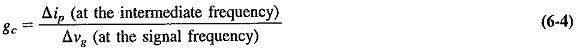The conversion transconductance of a transistor mixer is of the order of 6 mS, which is decidedly lower than the gm of the same transistor used as an amplifier. Since gc depends on the size of the local oscillator voltage, the above value refers to optimum conditions.

### Separately excited FET mixer:

In this circuit, which is shown in Figure 6-6, one device acts as a mixer while the other supplies the necessary oscillations. In this case, T1, the FET, is the mixer, to whose gate is fed the output of T2, the bipolar transistor Hartley oscillator. An FET is well suited for mixer duty, because of the square-law characteristic of its drain current. If T1 were a dual-gate MOSFET, the RF input would be applied to one of the gates, rather than to the source as shown here, with the local oscillator output going to the other gate, just as it goes to the single gate here. Note the ganging together of the tuning capacitors across the mixer and oscillator coils, and that each in practice has a trimmer (CTr) across it for fine adjustment by the manufacturer. Note further that the output is taken through a double-tuned transformer (the first IF transformer) in the drain of the mixer and fed to the IF amplifier. The arrangement as shown is most common at higher frequencies, whereas in domestic receivers a self-excited mixer is more likely to be encountered.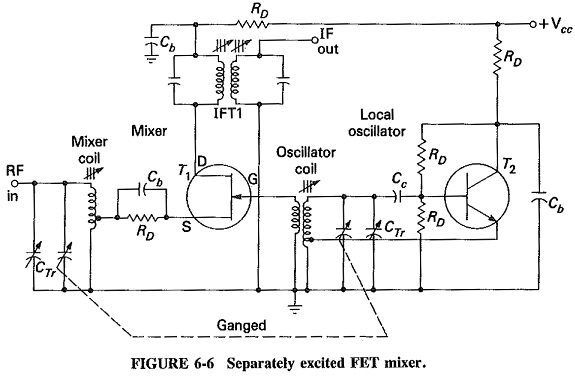### Self-excited Bipolar Transistor mixer:

The circuit of Figure 6-7 is best considered at each Frequency Changing in turn, but the significance of the L– L3 arrangement must first be explained. To begin, it is necessary that the tuned circuit L3 – CG be placed between collector and ground, but only for ac purposes. The construction of a ganged capacitor (CG is one of its sections) is such that in all the various sections the rotating plates are connected to one another by the rotor shaft. The rotor of the gang is grounded.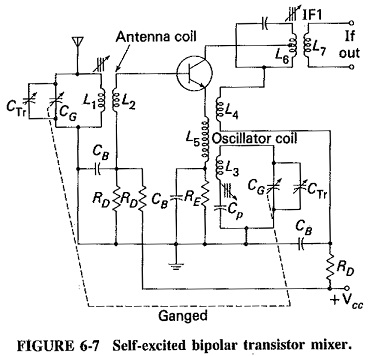One end of CG must go to ground, and yet there has to be a continuous path for direct current from HT to collector. One of the solutions to this problem would be the use of an RF choke instead of L4, and the connection of a coupling capacitor from the bottom of L6 to the top of L3. The arrangement as shown is equally effective and happens to be simpler and cheaper. It is merely inductive coupling instead of a coupling capacitor, and an extra transformer winding instead of an RF choke.

Now, at the signal Frequency Changing, the collector and emitter tuned circuits may be considered as being effectively short-circuited so that (at the RF) we have an amplifier with an input tuned circuit and an output that is indeterminate. At the IF, on the other hand, the base and emitter circuits are the ones which may be considered short-circuited. Thus, at the IF, we have an amplifier whose input comes from an indeterminate source, and whose output is tuned to the IF. Both these “amplifiers” are common-emitter amplifiers.

At the local oscillator Frequency Changing, the RF and IF tuned circuits may both be considered as though they were short-circuited, so that the equivalent circuit of Figure 6-8 results (at fo only). This is seen to be a tuned-collector Armstrong oscillator of the common-base variety.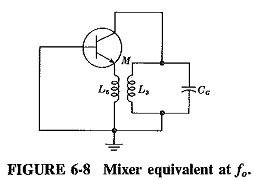We have considered each function of the mixer individually, but the circuit performs them all simultaneously of course. Thus, the circuit oscillates, the transcon­ductance of the transistor is varied in a nonlinear manner at the local oscillator rate and this variable gm is used by the transistor to amplify the incoming RF signal. Heterodyn­ing occurs, with the resulting production of the required intermediate Frequency Changing.

The superheterodyne receiver was developed to accomplish this as an improvement over some of the earlier attempts. This type of receiver incorporated some extra circuitry to ensure maximum signal reception (see Figure 6-9). Referring to the simplified block diagram in Figure 6-9, we can follow the signal process step by step.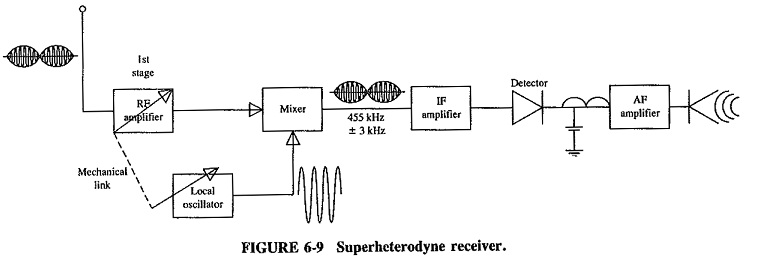The signal is received by the first-stage RF amplifier (which is a wideband class A amplifier) whose resonant Frequency Changing response curve can be tuned from 540 kHz to 1650 kHz (the standard broadcast band). The modulated signal is amplified and fed to the mixer stage (a class C circuit capable of producing the sum, difference, and original frequencies), which is receiving signals from two sources (the RF amplifier and the local oscillator). The unmodulated signal from the local oscillator is fed to the mixer simultaneously with the modulated signal from the RF amplifier (these two circuits are mechanically linked, as will be explained later in this section). The local oscillator (LO) is a tunable circuit with a tuning range that extends from 995 kHz to 2105 kHz.

The output from the mixer circuit is connected to the intermediate-frequency amplifier (IF amp), which amplifies a narrow band of select frequencies (455 kHz ± 3 kHz). In some receivers this class A circuit acts not only as an amplifier but also as a filter for unwanted frequencies which would interfere with the selected one. This new IF frequency contains the same modulated information as that transmitted from the source but at a frequency range lower than the standard broadcast band. This conversion process helps reduce unwanted interference from outside sources. The signal is rectified and filtered to eliminate one sideband and the carrier (conversion from RF to AF) and is finally amplified for listening.

#### To understand the process mathematically, follow these five steps:

1. The receiver is tuned to 550 kHz
2. The local oscillator (because of mechanical linking) will generate a frequency of 1005 kHz (always 455 kHz above the station carrier Frequency Changing)
3. The mixer will produce a usable output of 455 kHz (the difference frequency of LO — RF, 1005 kHz — 550 kHz)
4. The mixer output is fed to the IF amp (which can respond only to 455 kHz ± 3 kHz; all the other frequencies are rejected
5. The converted signal is rectified and filtered (detected), to eliminate the unusable portions, and amplified for listening purposes

This procedure is repeated for each station in the standard broadcast band and has proved to be one of the most reliable methods for receiving (over a wide band) without undue interference from adjacent transmitters.

The superheterodyne receiver (or any receiver for that matter) has a number of tunable circuits which must all be tuned correctly if any given station is to be received. The various tuned circuits are mechanically coupled so that only one tuning control and dial are required. This means that no matter what the received frequency, the RF and mixer input tuned circuits must be tuned to it. The local oscillator must simultaneously be tuned to a frequency precisely higher than this by the intermediate frequency. Any errors that exist in this frequency difference will result in an incorrect Frequency Changing being fed to the IF amplifier, and this must naturally be avoided. Such errors as exist are called tracking errors, and they result in stations appearing away from their correct position on the dial.

Keeping a constant frequency difference between the local oscillator and the front-end circuits is not possible, and some tracking errors must always occur. What can be accomplished normally is only a difference frequency that is equal to the IF at two preselected points on the dial, along with some errors at all other points. If a coil is placed in series with the local oscillator ganged capacitor, or, more commonly, a capacitor in series with the oscillator coil, then three-point tracking results and has the appearance of the solid curve of Figure 6-10.

The capacitor in question is called a padding capacitor or a padder and is shown (labeled Cp) in Figures 6-6 and 6-7. The wanted result has been obtained because the variation of the local oscillator coil reac­tance with Frequency Changing has been altered. The three frequencies of correct tracking may be chosen in the design of the receiver and are often as shown in Figure 6-10, just above the. bottom end of the band (600 kHz), somewhat below the top end (1500 kHz), and at the geometric mean of the two (950 kHz).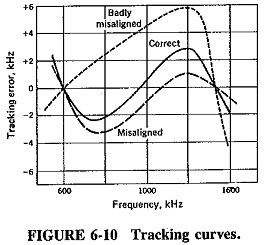It is entirely possible to keep maximum tracking error below 3 kHz. A value as low as that is generally considered quite acceptable. Since the padder has a fixed value, it provides correct three-point tracking only if the adjustable local oscillator coil has been preadjusted, i.e., aligned, to the correct value. If this has not been done, then incorrect three-point tracking will result, or the center point may disappear completely, as shown in Figure 6-10.

### Local Oscillator Frequency:

In receivers operating up to the limit of shortwave broadcasting, that is 36 MHz, the most common types of local oscillators are the Armstrong and the Hartley, The Colpitts, Clapp, or ultra-audion oscillators are used at the top of this range and above, with the Hartley also having some use if frequencies do not exceed about 120 MHz. Note that all these oscillators are LC and that each employs only one tuned circuit to determine its Frequency Changing. Where the frequency stability of the local oscillator must be particularly high, AFC (see Sections 5-3.3 and 6-3.2) or a frequency synthesizer (see Sections 6-3.3 and 6-5.2) may be used. Ordinary local oscillator circuits are shown in Figures 6-6 and 6-7.

The frequency range of a broadcast receiver local oscillator is calculated on the basis of a signal frequency range from 540 to 1650 kHz, and an intermediate frequency which is generally 455 kHz. For the usual case of local oscillator frequency above signal frequency, this range is 995 to 2105 kHz, giving a ratio of maximum to minimum frequencies of 2.2:1. If the local oscillator had been designed to be below signal frequency, the range would have been 85 to 1195 kHz, and the ratio would have been 14:1. The normal tunable capacitor has a capacitance ratio of approximately 10:1, giving a frequency ratio of 3.2:1. Hence the 2.2:1 ratio required of the local oscillator operating above signal frequency is well within range, whereas the other system has a frequency range that cannot be covered in one sweep. This is the main reason why the local oscillator frequency is always made higher than the signal frequency in receivers with variable-frequency oscillators.

It may be shown that tracking difficulties would disappear if the frequency ratio (instead of the frequency difference) were made constant. Now, in the usual system, the ratio of local oscillator frequency to signal frequency is 995/540 = 1.84 at the bottom of, the broadcast band, and 2105/1650 = 1.28 at the top of the band. In a local-oscillator-below-signal-frequency system, these ratios would be 6.35 and 1.38, respectively. This is a much greater variation in frequency ratio and would result in far more troublesome tracking problems.